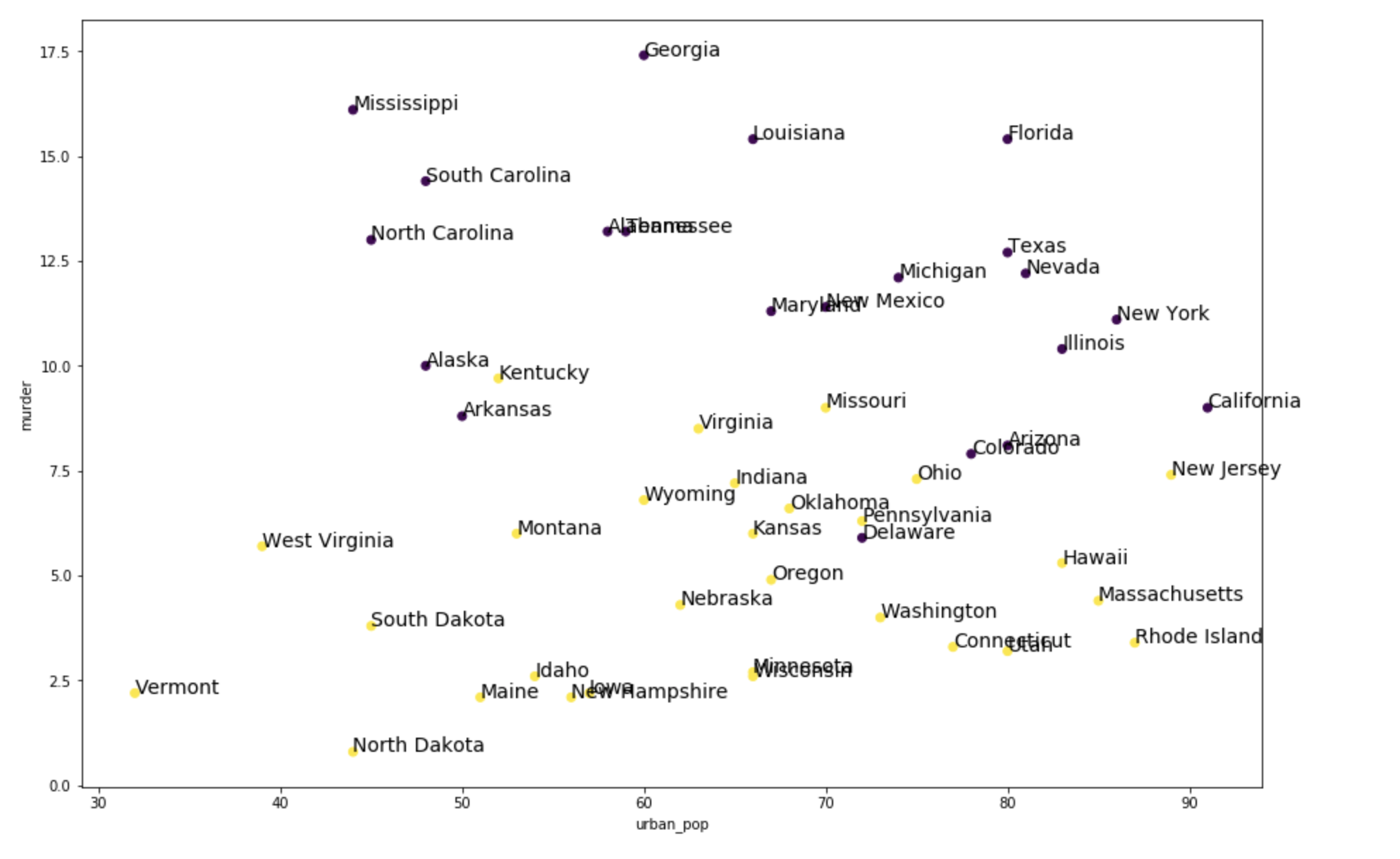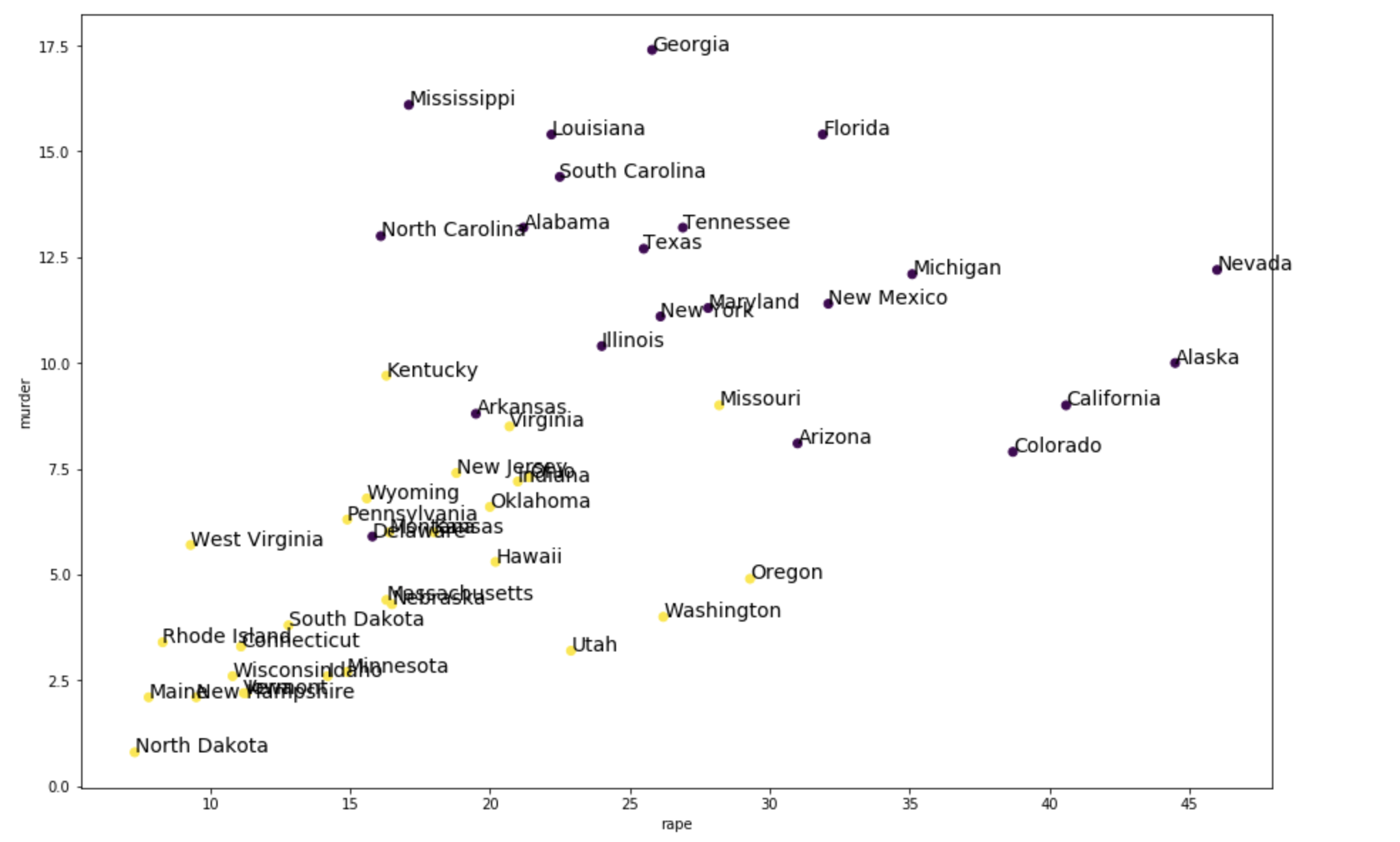# Cluster Using KMeans with Teradata Package for Python - 17.00 - Clustering Using KMeans with Teradata Package for Python - Teradata Package for Python

## Teradata® Package for Python User Guide

Product
Release Number
17.00
Published
November 2021
Language
English (United States)
Last Update
2022-01-14
dita:mapPath
bol1585763678431.ditamap
dita:ditavalPath
ayr1485454803741.ditaval
dita:id
rkb1531260709148
This example uses the US Arrests data of 50 samples containing statistics for arrests made per 100,000 residents for assault, murder, and rape in each of the 50 US states in 1973, along with the percentage of the population living in urban areas.

Example here shows how to use KMeans clustering function.

1. Import the required modules.
```from teradataml.analytics.mle.KMeans import KMeans
import matplotlib.pyplot as plt```
2. If the input table "kmeans_us_arrests_data" does not already exist, create the table and load the datasets into the table.
`load_example_data("kmeans", "kmeans_us_arrests_data")`
3. Create a teradataml DataFrame from "kmeans_us_arrests_data".
```## Creating TeradataML dataframes
df_train = DataFrame('kmeans_us_arrests_data')
```
```# Print train data to see how the sample train data looks like
4. In the training dataset, the features are 'sno', 'state', 'murder', 'assault', 'urban_pop' and 'rape'. And 'sno' to 'state' is a one-to-one mapping. For training, drop off the repeated feature 'state'.
```# A dictionary to get 'sno' to 'state' mapping. Required for plotting.
df1 = df_train.select(['sno', 'state'])
sno_to_state = dict(df1.to_pandas()['state'])
print(sno_to_state)
```
```# No need of 'state' columns, instead we have 'sno' column for the same
df_train = df_train.drop(['state'], axis=1)
colnames = ["sno", "murder", "assault", "urban_pop", "rape"]```
5. Apply KMeans algorithm to generate two clusters and inspect the outputs.
1. Apply KMeans algorithm.
```## KMmeans algorithm on data, with 2 centroids
kMeans_output = KMeans(data=df_train, centers=2, data_sequence_column=['sno'])
```
```# Print the KMeans results
print(kMeans_output)```
2. The 'cluster_centroids' output dataframe presents the centroid values of the two clusters, the withinness and the number of samples in each cluster out of all the samples.
```# Features (4-dimensional) used to train KMeans algorithm.
features = 'murder assault urban_pop rape'.split()
```
```# Feature Centroids for cluster 1
centroid_values = kMeans_output.clusters_centroids.to_pandas()['murder assault urban_pop rape'].split()
print("4-dimensional Centroid of Cluster 1:")
print(dict(zip(features, centroid_values)))
```
```# Feature Centroids for cluster 2
centroid_values = kMeans_output.clusters_centroids.to_pandas()['murder assault urban_pop rape'].split()
print("4-dimensional Centroid of Cluster 2:")
print(dict(zip(features, centroid_values)))```
Check the withinness to see if the within is the cluster sum of squares (from teradataml DataFrame output 'clusters_centroids').
```# Withinness for cluster 1
print("Withinness for cluster 1: " + str(kMeans_output.clusters_centroids.to_pandas()['withinss']))
```
```# Withinness for cluster 2
print("Withinness for cluster 2: " + str(kMeans_output.clusters_centroids.to_pandas()['withinss']))```
3. The 'clustered_output' dataframe presents the 'sno' representing state and the corresponding mapping number to cluster for all the samples.
`kMeans_output.clustered_output.head(30)`
4. The 'output' dataframe presents overall summary of the KMeans output.
`print(kMeans_output.output)`
6. Plot clusters based on feature to analyze the clustering output.
```## Inner join of clustered_output to actual dataset df_train We shall use the data from df1 to plot.
df1 = df_train.join(kMeans_output.clustered_output, how='inner', on=['sno'], lsuffix='t1',
rsuffix='t2')

print("\nInner join of clustered_output to actual dataset df_train:")
print(df1)```
1. Plot clusters based on the two features 'urban_pop' and 'murder'.
```# Selecting only the necessary features for plot.
df3 = df1.select(['t1_sno', 'urban_pop', 'murder', 'clusterid'])```
```# Since there is no plotting possible for teradataml DataFrame, we are converting it to
# pandas dataframe and then to numpy_array 'numpy_df' to use matplotlib library of python.
pandas_df = df3.to_pandas()
numpy_df = pandas_df.values
```
```# Setting figure display size.
plt.rcParams['figure.figsize'] = [15, 10]
```
```# Coloring based on cluster_id.
plt.scatter(numpy_df[:,1], numpy_df[:,2], c=numpy_df[:,3])
for ind, value in enumerate(numpy_df[:, 0]):
# sno_to_state is used hear to get state names.
plt.text(numpy_df[ind,1], numpy_df[ind,2], sno_to_state[int(value)], fontsize=14)
plt.xlabel('urban_pop')
plt.ylabel('murder')
plt.show()```
Run the above commands and the following plot shows:2. Plot the clusters based on the two features 'rape' and 'murder'.
```# Selecting only the necessary features for plot.
df3 = df1.select(['t1_sno', 'rape', 'murder', 'clusterid'])
```
```# Since there is no plotting possible for teradataml DataFrame, we are converting it to
# pandas dataframe and then to numpy_array 'numpy_df' to use matplotlib library of python.
pandas_df = df3.to_pandas()
numpy_df = pandas_df.values
```
```# Coloring based on cluster_id.
plt.scatter(numpy_df[:,1], numpy_df[:,2], c=numpy_df[:,3])
for ind, value in enumerate(numpy_df[:, 0]):
# sno_to_state is used hear to get state names.
plt.text(numpy_df[ind,1], numpy_df[ind,2], sno_to_state[int(value)], fontsize=14)
plt.xlabel('rape')
plt.ylabel('murder')
plt.show()```
Run the above commands and the following plot shows: